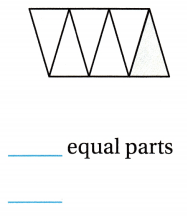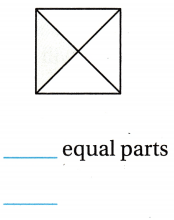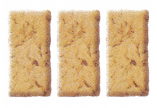Refer to our Texas Go Math Grade 3 Answer Key Pdf to score good marks in the exams. Test yourself by practicing the problems from Texas Go Math Grade 3 Module 2 Assessment Answer Key.

Vocabulary

Choose the best term from the box to complete the sentence.

Question 1.
A ___ is a number that names part of a whole or part of a group.
Numerator

Explanation:
A numerator is a number that names part of a whole or part of a group.

The ___ tells how many equal parts are in the whole or in the group.
Denominator

Explanation:
The denominator tells how many equal parts are in the whole or in the group.

Concepts and Skills

Write the number of equal parts in the whole. Then write the fraction in numbers that name the shaded part. TEKS 3.3.C

Question 3.3 equal parts
$$\frac{1}{3}$$

Explanation:
There are 3 equal parts in the whole of. The $$\frac{1}{3}$$ fraction in numbers that names the shaded part.

Question 4.6 equal parts
$$\frac{1}{6}$$

Explanation:
There are 6 equal parts in the whole of The $$\frac{1}{6}$$ fraction in numbers that names the shaded part.

Go Math Grade 3 Answer Key Pdf Module 2 Assessment Question 5.4 equal parts
$$\frac{1}{4}$$

Explanation:
There are 4 equal parts in the whole of. The $$\frac{1}{4}$$ fraction in numbers that names the shaded part.

Use fraction strips to help you complete the number line. Then locate and draw a point for the fraction. TEKS 3.3 A

Question 6.
$$\frac{1}{2}$$$$\frac{1}{2}$$

Explanation:Texas Test Prep

Fill in the bubble for the correct answer choice. You can use objects or models to solve.

Question 7.
Jessica ordered a pizza. What fraction of the pizza has mushrooms? TEKS 3.3.A(A) $$\frac{2}{6}$$
(B) $$\frac{2}{8}$$
(C) $$\frac{6}{6}$$
(A) $$\frac{8}{6}$$
$$\frac{2}{8}$$

Explanation:
The $$\frac{2}{8}$$ fraction of the pizza has mushrooms.

Go Math Module 2 Assessment Grade 3 Pdf Question 8.
Which is $$\frac{5}{6}$$ written as a sum of unit fractions? TEKS 3.3.D
(A) $$\frac{5}{6}$$ + $$\frac{5}{6}$$ + $$\frac{5}{6}$$ + $$\frac{5}{6}$$ + $$\frac{5}{6}$$
(B) $$\frac{1}{6}$$ + $$\frac{1}{6}$$ + $$\frac{1}{6}$$
(C) $$\frac{1}{6}$$ + $$\frac{1}{6}$$ + $$\frac{1}{6}$$ + $$\frac{1}{6}$$ + $$\frac{1}{6}$$ + $$\frac{1}{6}$$
(D) $$\frac{1}{6}$$ + $$\frac{1}{6}$$ + $$\frac{1}{6}$$ + $$\frac{1}{6}$$ + $$\frac{1}{6}$$
$$\frac{1}{6}$$ + $$\frac{1}{6}$$ + $$\frac{1}{6}$$ + $$\frac{1}{6}$$ + $$\frac{1}{6}$$

Explanation:
The $$\frac{5}{6}$$ written as a sum of unit fractions is $$\frac{1}{6}$$ + $$\frac{1}{6}$$ + $$\frac{1}{6}$$ + $$\frac{1}{6}$$ + $$\frac{1}{6}$$.

Question 9.
Joshua has 3 oatmeal bars to share with his 2 sisters and 3 of his friends. If he plans to give each person, including himself, an equal share, how much of an oatmeal bar will each person get? TEKS 3.3.E(A) 3 sixths
(B) 4 sixths
(C) 2 sixths
(D) 5 sixths
Joshua has 3 oatmeal bars to share with his 2 sisters and 3 of his friends. There are a total of 6 friends and he plans to give each person. Hence each person gets an oatmeal bar of $$\frac{3}{6}$$.
Elijah and Zoey each have an orange cut into 8 equal sections. They each ate one section, or $$\frac{1}{8}$$, of their orange. How many more sections altogether do Elijah and Zoey need to eat to finish both oranges? TEKS 3.3.C